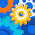## Sunday, 28 April 2013

### Forecasting stock returns using ARIMA model with exogenous variable in R

Why is it important?

# Why is it important?

India has a lot to achieve in terms of becoming a developed nation from an economic standpoint. An aspect which, in my opinion, is of utmost importance is the formation of structurally sound and robust financial markets. A prerequisite for that is active participation of educated and informed traders in the market place which would result in better price discovery and in turn better functioning market in general.

Statistical modelling techniques supplemented with some subject understanding could be an informed trading strategy. In the long run it might not be possible to outplay the market using a simple backward looking statistical model, but in the short run intelligent estimates based on model and subject matter expertise could prove to be helpful. In our previous posts with Infosys stock prices, we used basic visualization and simple linear regression techniques to try and predict the future returns from historical returns. Lets step on the pedal and move over to some more sophisticated techniques to do the same. We will start with the same basics of running basic checks on the data and then take a deeper dive in terms of modelling technique to use.

data <- read.csv("01-10-2010-TO-01-10-2011INFYEQN.csv")
summary(data)

##         Date      Close.Price
##  01-Apr-11:  1   Min.   :2183
##  01-Aug-11:  1   1st Qu.:2801
##  01-Dec-10:  1   Median :2993
##  01-Feb-11:  1   Mean   :2929
##  01-Jul-11:  1   3rd Qu.:3106
##  01-Jun-11:  1   Max.   :3481
##  (Other)  :245

plot(as.Date(data$Date, "%d-%b-%y"), data$Close.Price, xlab = "Dates", ylab = "Adjusted closing price",
type = "l", col = "red", main = "Adjusted closing price of INFOSYS for past 1 year")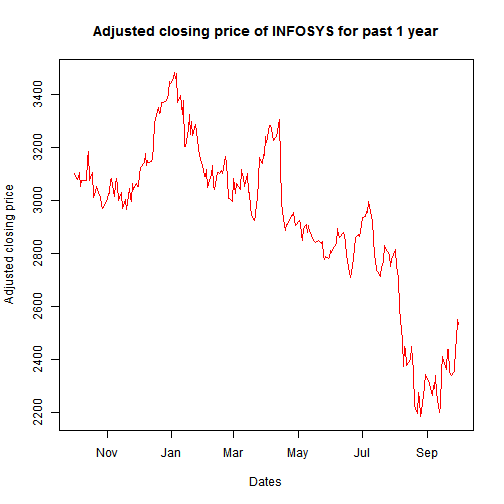There seems to be a lot of randomness in the series and the adf.test results prove that the series is non-stationary (I(1)). Which means that the series will have to be first differenced to make is stationary. (Refer to this post for more understanding on stationarity).

library(tseries, quietly = T)

adf.test(data$Close.Price)  ## ## Augmented Dickey-Fuller Test ## ## data: data$Close.Price
## Dickey-Fuller = -2.451, Lag order = 6, p-value = 0.3858
## alternative hypothesis: stationary

infy_ret <- 100 * diff(log(data$Close.Price))  # Auto-regressive moving average (ARMA) model There is one primary difference between time series and cross sectional datasets and that is the presence of auto-correlation in time series data. The concept of auto-correlation is not applicable to cross sectional regression as there is no dependence in the observations. However, there is explicit dependent of time series' future value on its near past values. We arrive at the estimates in a time series model after solving the Yule Walker equations unlike MLE or simple OLS techniques in the case of cross sectional linear regressions. The idea of an ARMA model is fairly intuitive to understand, however, the math gets extremely tricky. We will take a crack at explaining what an ARMA model is in laymen language. A typical ARMA(1,1) model can be expressed as : $\begin{equation} z_t = \alpha + \phi z_{t-1} + \theta\epsilon_{t-1} + \epsilon_t \end{equation}$ The (1,1) in the equation stand for the auto-regressive($$z_{t}$$) and moving average($$\epsilon_{t}$$) lag orders respectively. The intuitive understanding of the above equation is pretty straightforward. The current value of the time series $$z_t$$ will depend on the past value of the series $$z_{t-1}$$ and will correct itself to the error made in the last time period $$\epsilon_{t-1}$$. Lets try and fit an ARMA model to our INFY returns data and see how the results turn out. summary(arma(infy_ret, order = c(2, 2)))  ## ## Call: ## arma(x = infy_ret, order = c(2, 2)) ## ## Model: ## ARMA(2,2) ## ## Residuals: ## Min 1Q Median 3Q Max ## -9.5723 -0.9214 -0.0719 0.9289 4.5550 ## ## Coefficient(s): ## Estimate Std. Error t value Pr(>|t|) ## ar1 -0.34007 0.02591 -13.12 <2e-16 *** ## ar2 -0.97524 0.01817 -53.67 <2e-16 *** ## ma1 0.44427 0.00702 63.31 <2e-16 *** ## ma2 1.01522 0.00802 126.60 <2e-16 *** ## intercept 0.00525 0.21054 0.02 0.98 ## --- ## Signif. codes: 0 '***' 0.001 '**' 0.01 '*' 0.05 '.' 0.1 ' ' 1 ## ## Fit: ## sigma^2 estimated as 2.9, Conditional Sum-of-Squares = 718.3, AIC = 985.8  We can see that AR as well as MA coefficients are all significant at 99%, evident from the small p-values. Since our objective here is to forecast future returns lets evaluate the performance of the ARMA model in terms of out-of-sample forecast performance. For this we will divide the data into 2 parts, on one we will train the model and on the other we will test the out-of-sample forecast ability. Here Wehave used ARIMA function to fit the model as the object type “arima” is easily compatible with forecast() and predict() function. ARIMA is nothing by a normal ARMA model with the order of integration included as an argument to the function. In our case, our series was I(1) but we have first differenced it already so in the ARIMA function we will keep the “I” part = 0. library(forecast, quietly = T)  infy_ret_train <- infy_ret[1:(0.9 * length(infy_ret))] # Train dataset infy_ret_test <- infy_ret[(0.9 * length(infy_ret) + 1):length(infy_ret)] # Test dataset fit <- arima(infy_ret_train, order = c(2, 0, 2)) arma.preds <- predict(fit, n.ahead = (length(infy_ret) - (0.9 * length(infy_ret))))$pred
arma.forecast <- forecast(fit, h = 25)

plot(arma.forecast, main = "ARMA forecasts for INFY returns")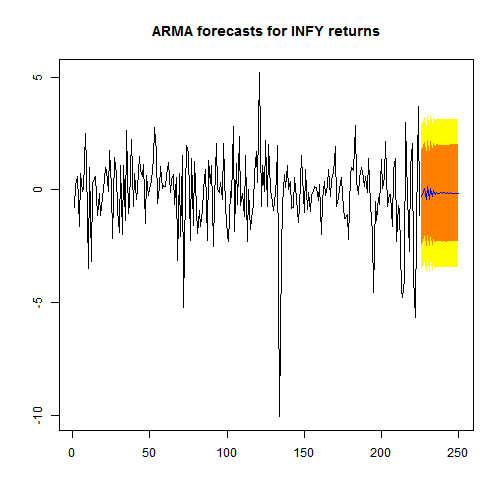accuracy(arma.preds, infy_ret_test)  # RMSE values

##  RMSE
## 2.489


Above are the results that we obtain with a simple ARMA(2,2) model. The orange and yellow region provide us the 99% and 95% confidence level for the forecasts respectively. An intrinsic shortcoming of ARMA models, which is evident from the plot above, is the assumption of mean reversion of the series. What this means is that after some time in future the forecasts would tend to the mean of the time series $$z_{t}$$'s historical values thus making it a poor model for long term predictions.

Now, there are some intuitive variables that one can introduce in the model based on subjective understanding to improve the model. In cases where one wishes to augment a simple univariate time series regression with some exogenous set of variable, ARIMAX function can be employed. In cases where the additional variables could have a feedback relation with the time series in question (i.e they are endogenous) one can employ Vector auto regressive (VAR) models. Let me try and elaborate a little on them before I start to sound confusing. In our example above in question, lets say that our hypothesis is that day of the week has an effect on the stock prices. To include this in our model all that we need is 4 new dummy variables for 4 days of the week (5th one by default goes to the intercept) and include them in the above ARMA model using ARIMAX function. Here these dummy variables will be completely exogenous to our dependent variable (INFY returns), because no matter how/what the stock price is for INFY, its not going to affect the day of the week! However, lets say we wanted to include NIFTY returns as an additional variable in the analysis, a VAR model would be preferable. The reason being that there could be a feedback relation between INFY returns and NIFTY returns which might be ignored if we use a simple ARIMAX function.

# ARIMA model with day of the week variable

We will try and illustrate with an example the former where we will use day of the week as an exogenous variable to augment our ARMA model for INFY returns. The ARIMAX model can be simply written as:

$\begin{equation} z_t = \alpha + \phi z_{t-1} + \theta\epsilon_{t-1} + \gamma x_t + \epsilon_t \end{equation}$

where, $$x_{t}$$ is the exogenous variable. In our case we will have 4 dummy variables created for the 4 days.

data$day <- as.factor(weekdays(as.Date(data$Date, "%d-%b-%y")))
days <- data$day[2:nrow(data)] xreg1 <- model.matrix(~as.factor(days))[, 2:5] colnames(xreg1) <- c("Monday", "Thursday", "Tuesday", "Wednesday") fit2 <- arima(infy_ret_train, order = c(2, 0, 2), xreg = xreg1[c(1:(0.9 * length(infy_ret))), ]) fit1.preds <- forecast(fit2, h = 25, xreg = xreg1[c(226:250), ]) fit1.preds <- predict(fit2, n.ahead = 25, newxreg = xreg1[c(226:250), ]) plot(forecast(fit2, h = 20, xreg = xreg1[c(226:250), ]), main = "ARIMAX forecasts of INFY returns")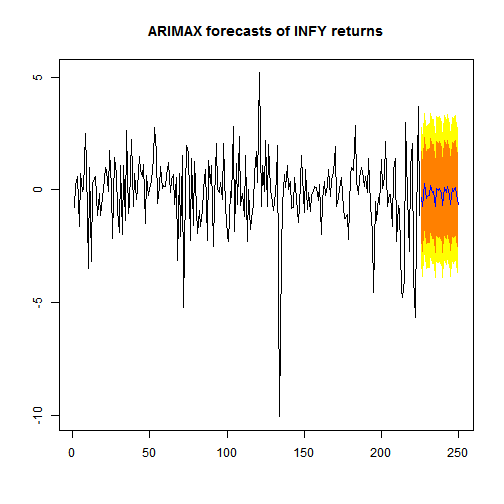accuracy(fit1.preds$pred, infy_ret_test)

##  RMSE
## 2.431


The performance of the ARIMA model with weekdays factor variable seems to be better than a simple ARMA model which is evident from the lower RMSE of the ARIMAX model. This is just one example of variables that could be used to augment a simple ARMA model, there could be many more variants of such variables that might further increase the performance of the model. In the next post we would try to cover vector auto regression and how/when it can be used.

Feedback/criticisms welcome.

1.Hey where did you get the data '01-10-2010-TO-01-10-2011INFYEQN.csv' from ? Please if you could help in letting me know that.

1.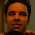Sorry for the delayed response. This data was obtained from PROWESS database.

Thanks,
Shreyes

2.If you can't subscribe to paid databases, you can use yahoo finance to get the stock data and oanda for fx data.
http://www.r-bloggers.com/financial-data-accessible-from-r-part-iv/

2.Human traders based on their experience in terms of stock price patterns, volume changes, and market news/rumors regarding a particular stock.

Stock Forecast

3.so good post.I appricate to read this post.Thank you so much for sharing.
stock forecast

4.How did you find the desired transformation required before hand ?
Why have you opted for "infy_ret <- 100 * diff(log(data$Close.Price)) " ? 1.This transformation is the "thumb rule" transformation applied to non-stationary (I(1)) series to make them stationary. Log was simply taken to scale down the price values and the first difference was taken to remove the non-stationarity. 5.Just want to point out: you can't fit a arma(n,n) model as it doesn't make any sense in any physical system. It has to be arma(n,m) with n>m (strictly), otherwise your results are spurious. 6.exceptional article on," how forecasting on return on stocks can be done with the use of ARIMA model???..." and consequently gaining best stock signals. 7.I didn't know that deriving the stock tips is such a complicated and tricky process. 8.I think that what you did in the second part of the example is a regression with ARMA errors, not an ARMAX model. If you look at the ?arima help file in R, you can see in the details of the function this: "If an xreg term is included, a linear regression (with a constant term if include.mean is true and there is no differencing) is fitted with an ARMA model for the error term.", so if I understand well, you actually fitted a linear regression with an ARMA(2,2) on the errors. 1.I think the confusion is arising because I have used the term "ARIMAX" loosely. What I have done in the second part of the post is just an ARIMA model with an exogenous variable which, I have wrongly, termed as "ARIMAX" regression in some parts of the post. ARIMAX regression is different ball game altogether. Thanks for pointing this out Patricia. 9.how can we predict close price for future day i mean for jan2014 to dec 2013 10.11.Seriously, it great way of getting stock returns using ARIMA model. I read post shared by you. You explained very well. I will certainly consider it. Thank you so much for informative post on stock! best online trading platforms 12.I have one question on that..... Did you get the parameter value for those dummy variables which you created which you create in fit2 13.> arma <- forecast(fit, h = 25) Error: could not find function "forecast" "I am getting this error" .. can you please help me ?? 14.Now that you just acknowledge a number of tips on Forex, you'll be able to either get your feet wet or come back to into the sport armed with new information. Apply what you've got scan throughout this text and you are sure to be making higher trades and exchanges, in no time within the slightest degree. To know more forex signals 15.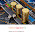Trading of foreign currency or Forex trading as it is known is an easy way to make huge money by investing a small amount. There have been many traders who have, made huge profits by using the expert advice from others who have been on the forex platform for many years. Number of reasons makes Forex market the best place for investors who like their money to grow quickly. The highest daily turnover sure makes the Forex market the best place for any new trader to get accustomed with ups and downs of stock market. To know more about forex signals trading. You visit and join us: forex signals 16.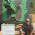Hallo, As I am new to stocks, just wanted to know how to Analyse the data. ARIMA models helps us to predict but what for Analysis..? 17.Beginners of the commerce must be made aware that forex trading is a excessive danger investment. The foreign money market gives a number of alternative to earn big amounts of earnings however at the identical time coupled with loads of risks. Foreign money buying and selling can provide you a fortune in minutes, days and hours. However the sad truth is that it can be misplaced simply on the identical time. To know more visit forex signals 18.How to predict future gas price using Forecasting in machine learning? 19.Do you need daily trading signals with trading forecast? you should follow this website to get 100% free profitable forex signals. You are invited to come on this website. 20.ForexFunction is providing one of the best trading signal service in the world. They have daily analysis section, trading idea, live signals, profitable strategy and much more. They have Trade copier service with free profitable forex signals. Come and join with this website. 21.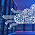Best Windows Forex VPS for EA Trading STANDARD VPS Plan 1GB Dedicated RAM 2X18GB SSD Raid 2 Core CPU$6.90/Month

22.23.